﻿ PDST Post-Primary Maths | Learn to use calculators
Working together to improve teaching, learning and assessment Leagan Gaeilge
For students ›› Learn to use calculators

## Get the most from your calculator

Below are a vast array of instructional materials designed to help students understand their calculator and become practiced at carrying out various calculations.

### New Resources for Casio fx-83GT

Correlation Coefficient on fx-83GT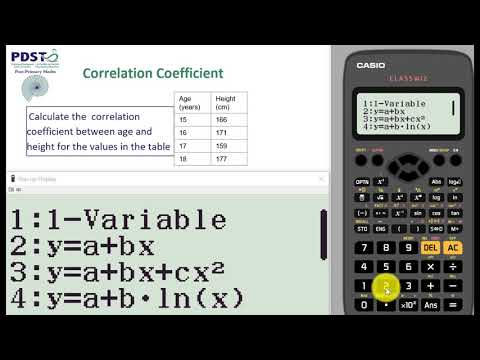Calculate correlation coefficient on your Casio fx-83GT calculator

Standard Deviation from a List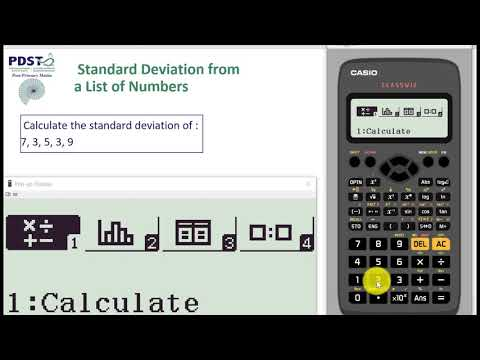Calculate standard deviation from a list of numbers on your Casio fx-83GT calculator

Standard Deviation from a Frequency Tabl...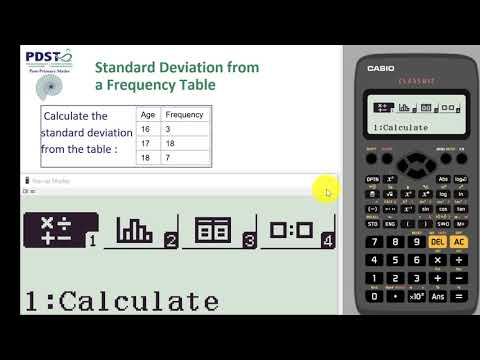Calculate standard deviation from a frequency table on your Casio fx-83GT calculator

### Calculator Instructional Videos

Lowest Common Multiple (LCM)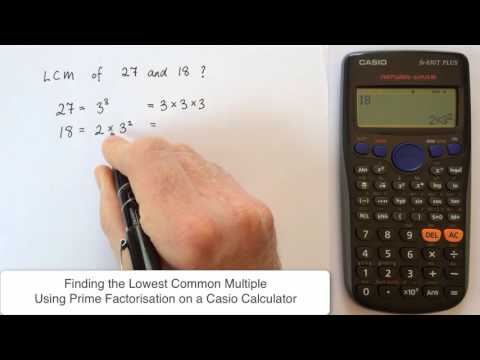Learn to use your calculator to calculate LCM

Prime Factors & HCF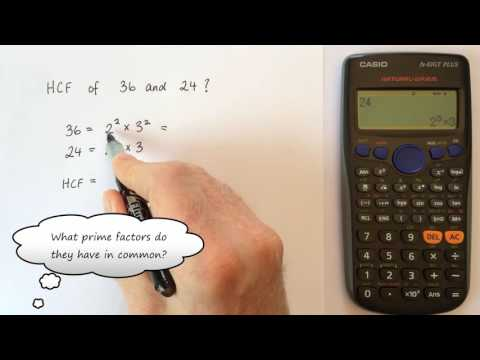Learn to find prime factors and find HCF

Table Function for Graphing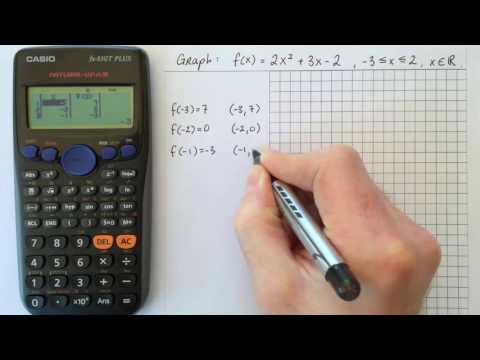Use your calculator to find couples for plotting a graph

Combinations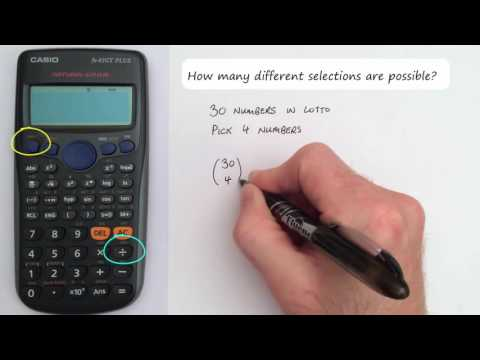Learn to calculate the number of combinations

Standard Deviation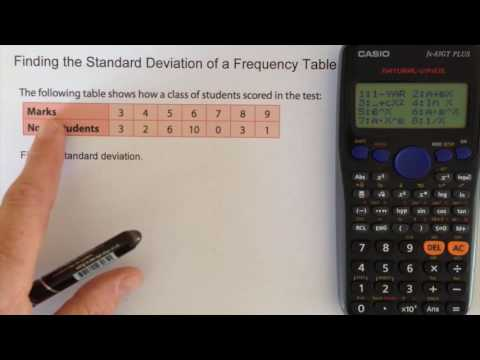Learn to calculate standard deviation

Correlation Coefficient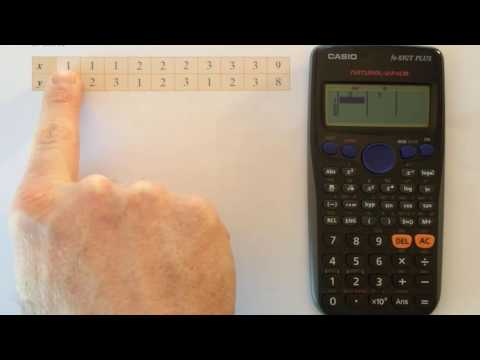Learn to calculate correlation coefficient

Logarithms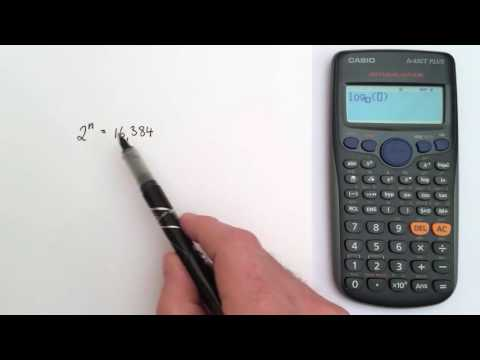Learn to use your calculator to calculate logs

Verify Mode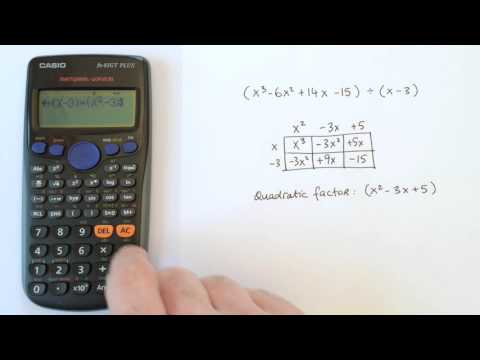Learn to verify solutions on your calculator & see where it's useful in the classroo...

### Why Use Calculators

Used appropriately, calculators are an invaluable tool in today’s maths classroom. They can be used to promote deeper understanding of many maths concepts for example in investigating the properties of numbers or in speeding up rote calculations as part of a much deeper learning activity. It is important that students are proficient in using their calculators and it is also important that students understand the calculations they are doing rather than blindly keying in figures. Students should be encouraged to develop checking strategies so that they have some way of recognising an answer as being reasonable or not. Here is a brief overview of the importance of calculators to students’ maths education.